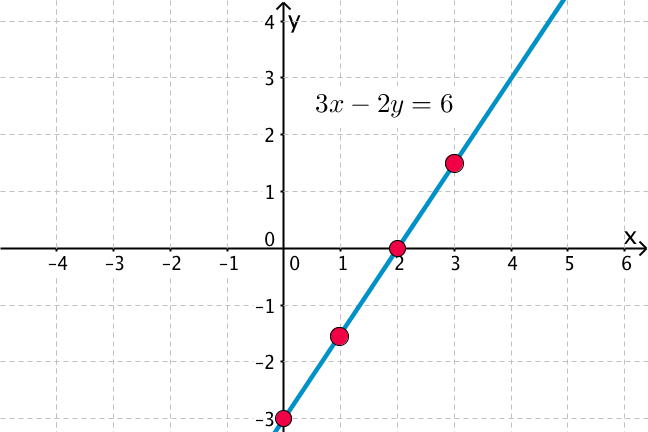# Solve this: Q. 2x + 3y = 17 3x - 2y = 6

Dear student,

Regards

• 1
2x + 3y =17
3x - 2y = 6

Rearranging 2x + 3y =17
2x = 17 - 3y
x = (17  - 3y)/2

Now replacing x with  (17  - 3y)/2  in 3x - 2y = 6
3{ (17  - 3y)/2 }  -2y  = 6
(51/2) - (9/2)y -2y = 6
-(13/2)y  = 6 -  51/2 = -39/2
y = 3
x  = (17  - 3y)/2  = ( 17 -9)/2 = 8/2 = 4
x=4, y = 3
• -1These are the graphs for these equations.
A friend above has given the solutions for the equations.
• 0
What are you looking for?